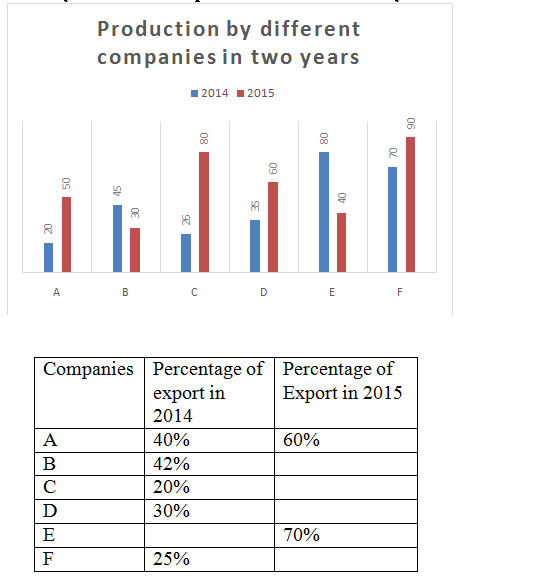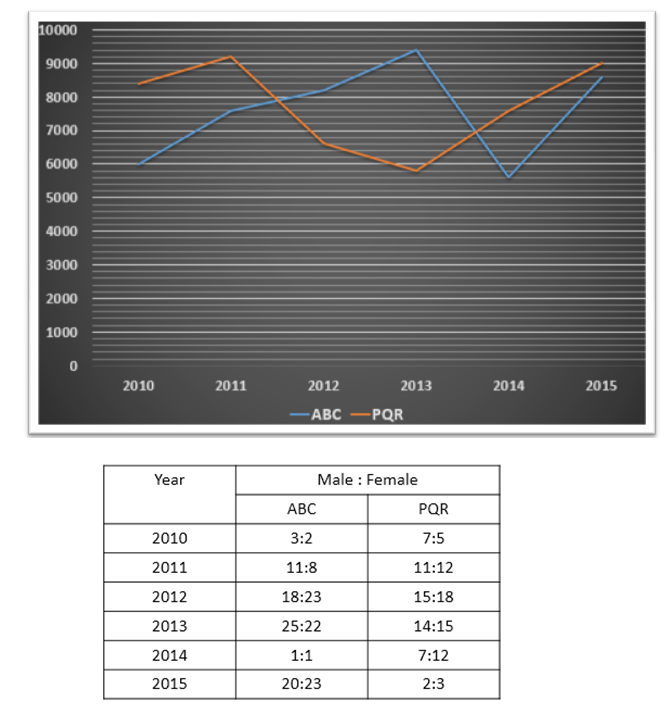# Quantitative Aptitude Questions (Data Interpretation) for SBI Clerk 2018 Day-38

## Quantitative Aptitude Questions (Data Interpretation) for SBI Clerk 2018 Day-38:

Dear Readers, SBI is conducting Online preliminary Examination for the recruitment of Clerical Cadre. preliminary Examination of SBI Clerk was scheduled from June/July 2018. To enrich your preparation here we have providing new series of Data Interpretation – Quantitative Aptitude Questions. Candidates those who are appearing in SBI Clerk Prelims Exam can practice these Quantitative Aptitude average questions daily and make your preparation effective.

Click “Start Quiz” to attend these Questions and view Solutions

## Daily Practice Test Schedule | Good Luck

 Topic Daily Publishing Time Daily News Papers & Editorials 8.00 AM Current Affairs Quiz 9.00 AM Logical Reasoning 10.00 AM Quantitative Aptitude “20-20” 11.00 AM Vocabulary (Based on The Hindu) 12.00 PM Static GK Quiz 1.00 PM English Language “20-20” 2.00 PM Banking Awareness Quiz 3.00 PM Reasoning Puzzles & Seating 4.00 PM Daily Current Affairs Updates 5.00 PM Data Interpretation / Application Sums (Topic Wise) 6.00 PM Reasoning Ability “20-20” 7.00 PM English Language (New Pattern Questions) 8.00 PM General / Financial Awareness Quiz 9.00 PM

Direction (Q. 1- 5) The graph below show production of mobiles (‘000) units by different companies in two different years:1. The average number of mobiles exported by company D and F in 2015 is 35000 while the mobiles exported by company D in 2014 is twice the export in 2015 then find percentage of export by company F out of total production of company F in 2015?

a) 67%

b) 72%

c) 80%

d) 67%

e) 87%

1. If company E exported a total of 50000 mobiles in the year 2014 and 2015 and the ratio between the export in 2014 and 2016 is 11: 5 then find the number of mobiles produced by company E in 2016 if export is 80% of the production?

a) 11120

b) 12500

c) 19890

d) 10250

e) 12750

1. The total number of mobiles exported by company A in 2014 and 2015 is 2/3 of the mobiles exported by company C in these years then find the percentage of mobiles that were exported by company C out of total production in 2015 ?

a) 90.25%

b) 67.5%

c) 25.75%

d) 64.75%

e) 56.5%

1. The 20% of the mobiles exported by company B in 2014 are defective while the ratio between the defective mobiles exported by company B in the year 2014 and 2015 is 7:5 and the percentage of defective mobiles out of total export by company B in 2015 is 13.5% then find the percentage of mobile exported by company B out of total production?

a) 45.66%

b) 67.77%

c) 52.65%

d) 66.67%

e) 56.15%

1. The mobiles exported by company A, B and D in 2014 is what percent the export by A and E in 2015?

a) 45%

b) 67%

c) 65%

d) 66%

e) 56%

Directions (Q. 6 – 10): Study the following information carefully and answer the questions given below:

The following graph represents number of employees working in 2 different companies in six years.1. Find the respective ratio of total number of males in ABC in 2010 and 2011 together and total number of females in PQR in 2012 and 2013 together.

a) 38:39

b) 40:33

c) 39:38

d) 33:44

e) none of these

1. Number of employees in ABC in 2012, 2013 and 2014 together is what percent of number of employees in PQR in 2010, 2011 and 2015 together?

a) 87.21%

b) 58.23%

c) 77.22%

d) 67.25%

e) none of these

1. Find the sum of total number of males in ABC in all the years together.

a) 24500

b) 18800

c) 23400

d) 15600

e) none of these

1. Find the difference between total number of females in ABC in all the years together and total number of females in PQR in all the years together.

a) 3100

b) 5800

c) 4200

d) 3500

e) none of these

1. Total number of males in PQR in all the years together forms what percent of total number of employees in PQR in all the years together?

a) 44.11%

b) 48.12%

c) 43.23%

d) 46.13%

e) none of these

Direction (Q. 1- 5)

Total mobiles exported by company D and F= 35000*2= 70000

Mobile exported by company D in 2014= 30/100* 35000= 10500

Mobile exported by company D in 2015= 10500/2= 5250

So,

Mobile exported by company F= 70000- 5250= 64750

Percentage of production exported by company F in 2015

= 64750/90000* 100

= 72%

Mobile exported by company E in 2015= 40000*70/100= 28000

Mobile exported by company E in 2014= 50000- 28000= 22000

Mobile exported by company E in 2016= 22000/11* 5= 10000

Number of mobiles produced by company E= 10000/80* 100= 12500

Total number of mobiles exported by company A in 2014 and 2015

= 40/100* 20000 + 60/100* 50000

= 8000 + 30000

= 38000

Mobile exported by company C in 2014 and 2015= 38000 *3/2= 57000

Mobile exported by company C in 2015

= 57000 – (26000* 20/100)

= 51800

So,

Percentage of export by company C

= 51800/80000* 100

= 64.75 %

Number of defective mobile exported by B in 2014 = 45000*42/100* 20/100=3780

Number of defective mobile exported by company B in 2015= 3780/7* 5= 2700

Number of mobiles exported by company B= 2700/13.5 * 100= 20000

So,

Percentage of mobile exported by company B in 2015= 20000*100/30000= 66.66%

Mobile exported by A, B and D in 2014

= 40*20000/100 + 42* 45000/100 + 30* 35000/100

= 8000 + 18900 + 10500

= 37400

Mobile exported by company A and E in 2015

= 50000*60/100 + 70*40000/100

= 30000 + 28000

= 58000

Required percentage

= 37400*100/ 58000

=65%

Directions (Q. 6 – 10):

Total number of males in ABC in 2010 and 2011 together

= 3/5 x 6000 + 11/19 x 7600

= 3600 + 4400

= 8000

Total number of females in PQR in 2012 and 2013 together

= 18/33 x 6600 + 15/29 x 5800

= 3600 + 3000

= 6600

Required ratio = 8000 : 6600 = 40:33

Number of employees in ABC in 2012, 2013 and 2014 together = 8200 + 9400 + 5600 = 23200

Number of employees in PQR in 2010, 2011 and 2015 together = 8400 + 9200 + 9000 = 26600

Required percentage = 23200/26600 x 100 = 87.21%

Total number of males in ABC in all the years together

= 3/5 x 6000 + 11/19 x 7600 + 18/41 x 8200 + 25/47 x 9400 + ½ x 5600 + 20/43 x 8600

= 3600 + 4400 + 3600 + 5000 + 2800 + 4000

= 23400

Total number of females in ABC in all the years together

= 2/5 x 6000 + 8/19 x 7600 + 23/41 x 8200 + 22/47 x 9400 + ½ x 5600 + 23/43 x 8600

= 2400 + 3200 + 4600 + 4400 + 2800 + 4600

= 22000

Total number of females in PQR in all the years together

= 5/12 x 8400 + 12/23 x 9200 + 18/33 x 6600 + 15/29 x 5800 + 12/19 x 7600 + 3/5 x 9000

= 3500 + 4800 + 3600 + 3000 + 4800 + 5400

= 25100

Required difference = 25100 – 22000 = 3100

Total number of males in PQR in all the years together

= 7/12 x 8400 + 11/23 x 9200 + 15/33 x 6600 + 14/29 x 5800 + 7/19 x 7600 + 2/5 x 9000

= 4900 + 4400 + 3000 + 2800 + 2800 + 3600

= 21500

Total number of employees in PQR in all the years together

= 8400 + 9200 + 6600 + 5800 + 7600 + 9000

= 46600

Required percentage = 21500/46600 x 100 = 46.13%